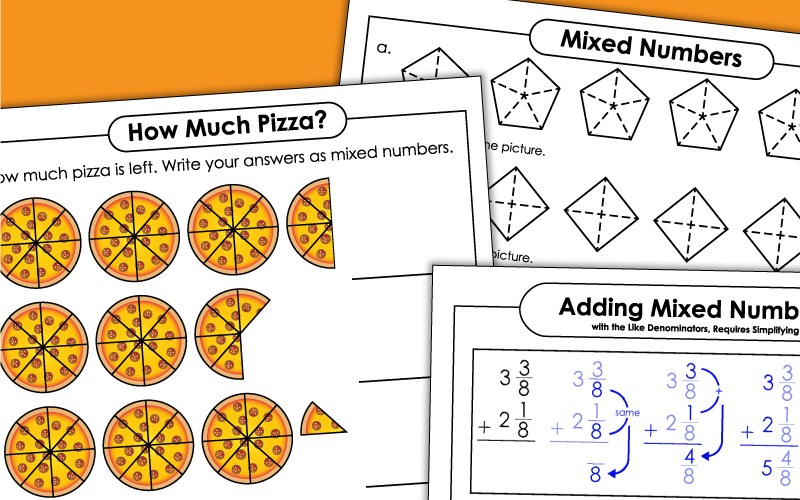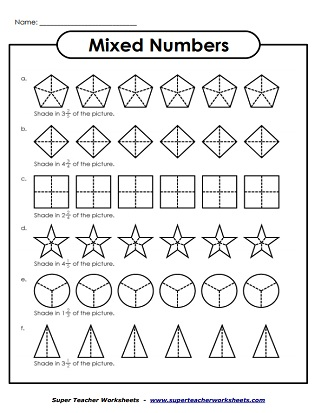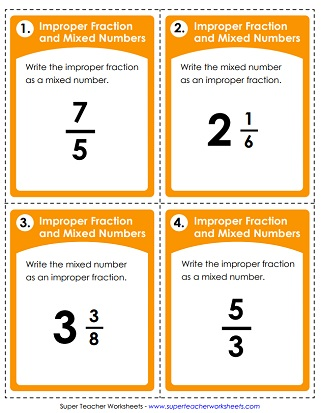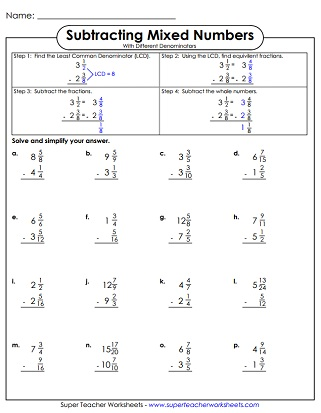# Mixed Numbers & Improper Fractions

This is a collection of improper fraction and mixed number worksheets, including worksheets on adding and subtracting mixed numbers.## Mixed Numbers(Basic Concept)

Mixed Numbers  FREE
Students write the mixed number shown by each illustration.
Students color the sets of shapes to show a mixed number.

## Improper Fractions andMixed Numbers

Convert each mixed number into an improper fraction and vice-versa. There's also a word problem on this page.
Write the correct mixed number and improper fraction for each illustration.
Write improper fractions as mixed numbers, and vice-versa. This set includes 30 task cards and an answer sheet. Use these cards for learning centers, small group instruction, classroom games, exit slips, or scavenger hunts.
Write the mixed number for each fraction and vice-versa; tenths
Cut out each improper fraction and glue it next to the correct mixed number. This version has denominators equal to 5 or less.
Cut out each mixed number and glue it next to the correct improper fraction. This version is harder, with denominators up to 12.

## Ordering and ComparingMixed Numbers

Place the mixed numbers in order on the number line.  Then use comparing symbols to determine the greater number.

Add the mixed numbers with like denominators; Vertical problems; Requires simplifying
A dozen mixed number addition problems.  Denominators are the same.
Add the mixed numbers with different denominators. Most sums have improper fractions. Some addends are improper as well.
Help students practice a range of different types of mixed-number-addition problems with this variety worksheet.
Use this worksheet to help students practice their mixed-number addition skills.
Subtract the mixed numbers with the same denominator; Vertical problems; Requires simplifying; Includes a word problem
Use this worksheet to help students practice a variety of styles of mixed-number-subtraction problems.
Practice solving several different types of mixed number subtraction problems with this variety worksheet.

Fraction Worksheets

This page has worksheets for teaching basic fraction skills, equivalent fractions, simplifying fractions, and ordering fractions. There are also links to fraction and mixed number addition, subtraction, multiplication, and division.

Decimal Worksheets

There are more mixed number skills on the decimal worksheets page

## Sample Worksheet ImagesMy Account
Site Information